Скачать презентацию Algorithms for Constraint Satisfaction in User Interfaces Scott

c1c995b6581e5516f0cfdf72b13bf06a.ppt

• Количество слайдов: 71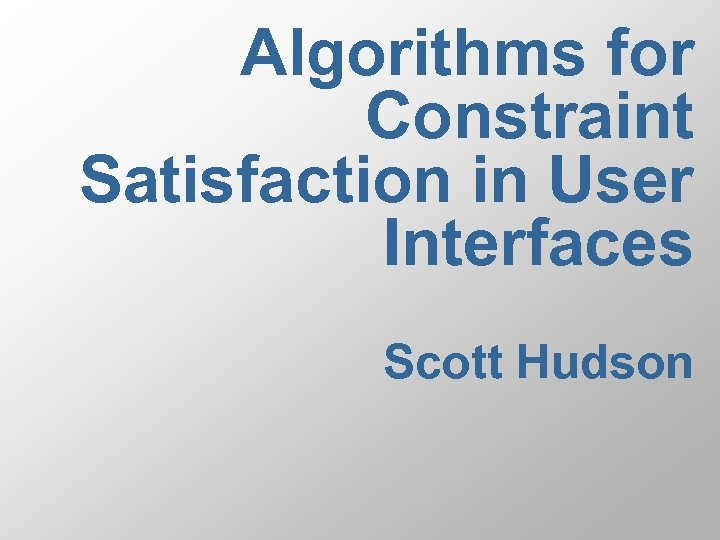Algorithms for Constraint Satisfaction in User Interfaces Scott Hudson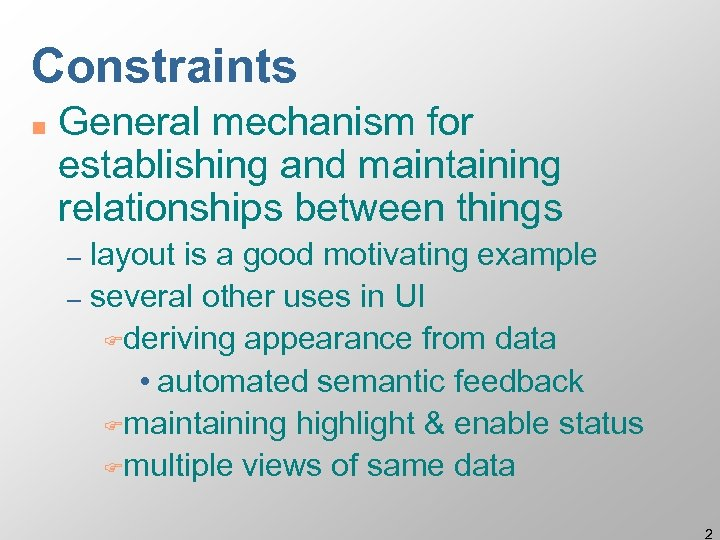Constraints n General mechanism for establishing and maintaining relationships between things layout is a good motivating example – several other uses in UI Fderiving appearance from data • automated semantic feedback Fmaintaining highlight & enable status Fmultiple views of same data – 2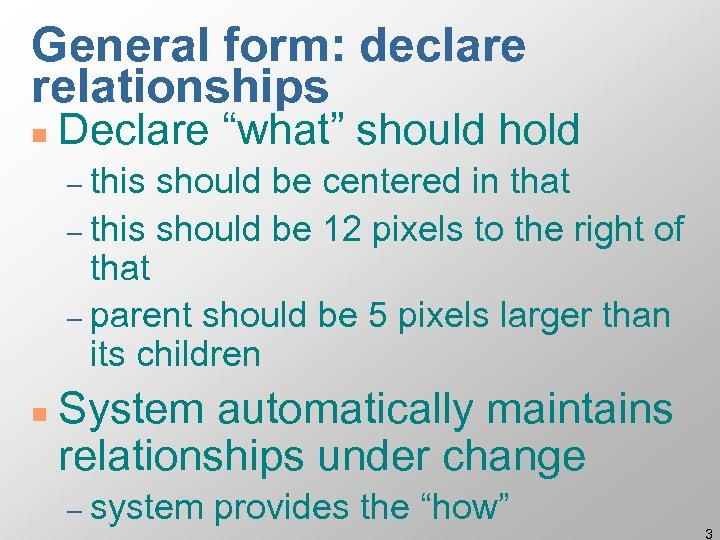General form: declare relationships n Declare “what” should hold – this should be centered in that – this should be 12 pixels to the right of that – parent should be 5 pixels larger than its children n System automatically maintains relationships under change – system provides the “how” 3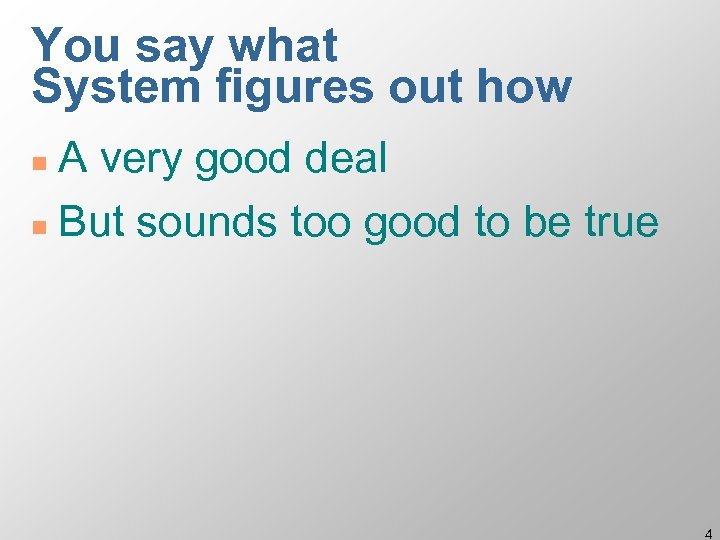You say what System figures out how A very good deal n But sounds too good to be true n 4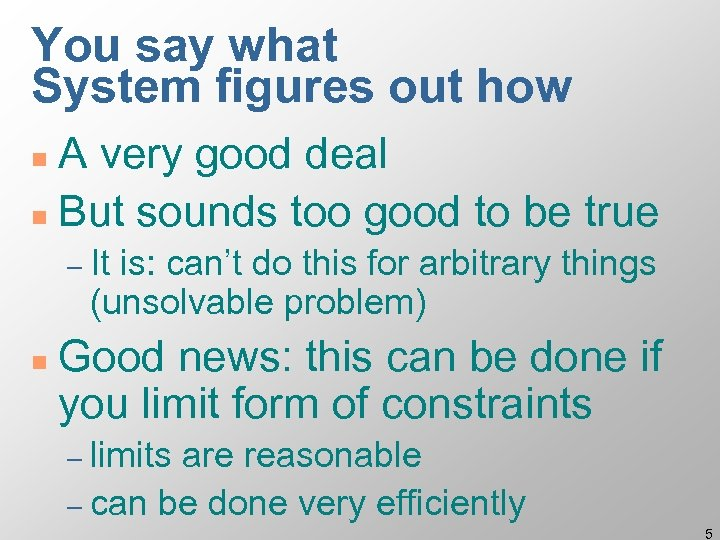You say what System figures out how A very good deal n But sounds too good to be true n – It is: can’t do this for arbitrary things (unsolvable problem) n Good news: this can be done if you limit form of constraints – limits are reasonable – can be done very efficiently 5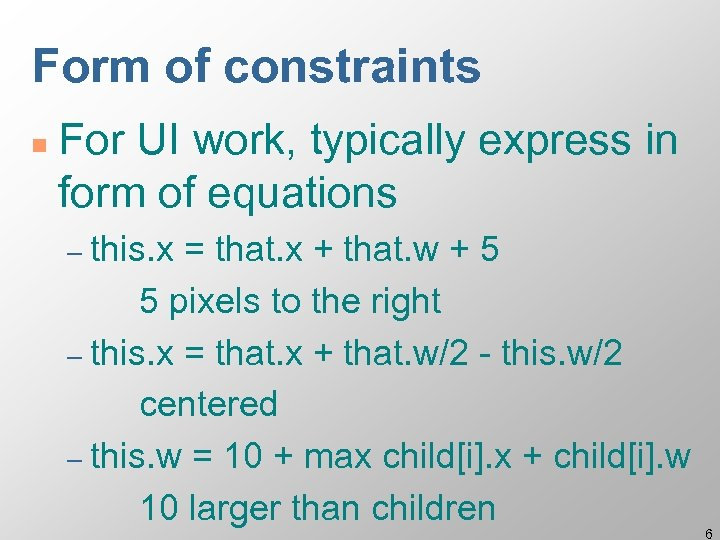Form of constraints n For UI work, typically express in form of equations – this. x = that. x + that. w + 5 5 pixels to the right – this. x = that. x + that. w/2 - this. w/2 centered – this. w = 10 + max child[i]. x + child[i]. w 10 larger than children 6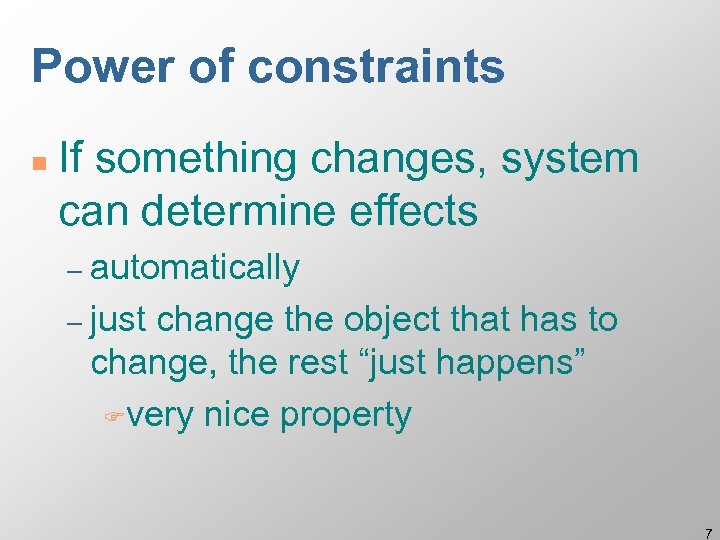Power of constraints n If something changes, system can determine effects – automatically – just change the object that has to change, the rest “just happens” Fvery nice property 7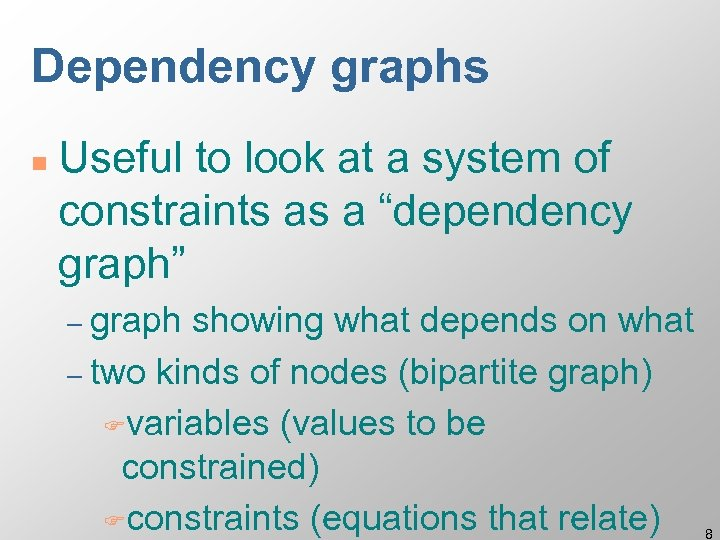Dependency graphs n Useful to look at a system of constraints as a “dependency graph” – graph showing what depends on what – two kinds of nodes (bipartite graph) Fvariables (values to be constrained) Fconstraints (equations that relate) 8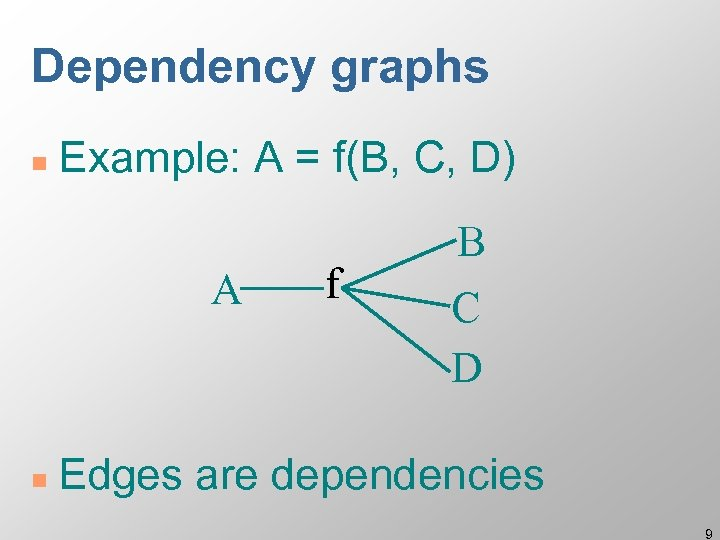Dependency graphs n Example: A = f(B, C, D) A n f B C D Edges are dependencies 9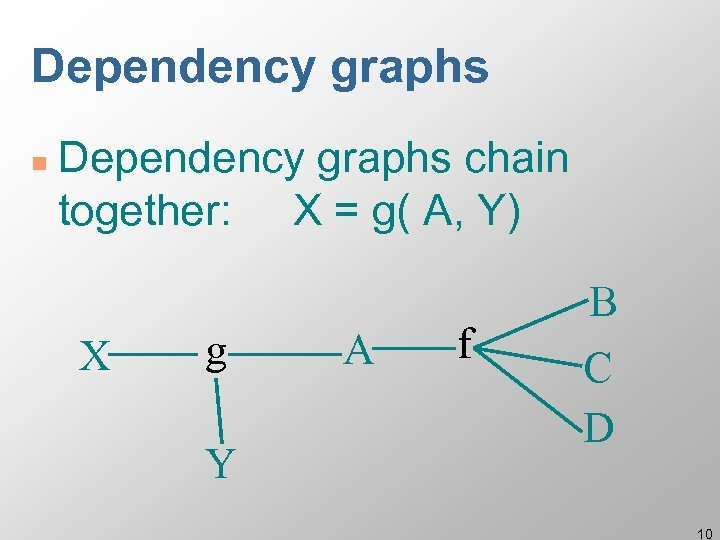Dependency graphs n Dependency graphs chain together: X = g( A, Y) X g Y A f B C D 10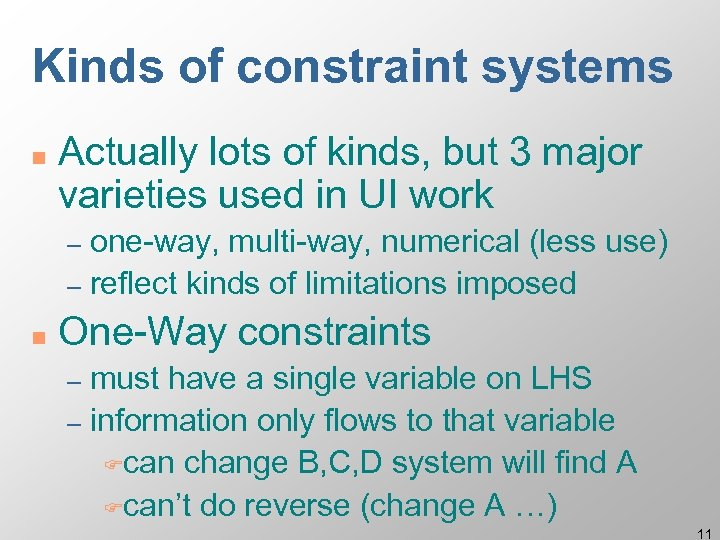Kinds of constraint systems n Actually lots of kinds, but 3 major varieties used in UI work one-way, multi-way, numerical (less use) – reflect kinds of limitations imposed – n One-Way constraints must have a single variable on LHS – information only flows to that variable Fcan change B, C, D system will find A Fcan’t do reverse (change A …) – 11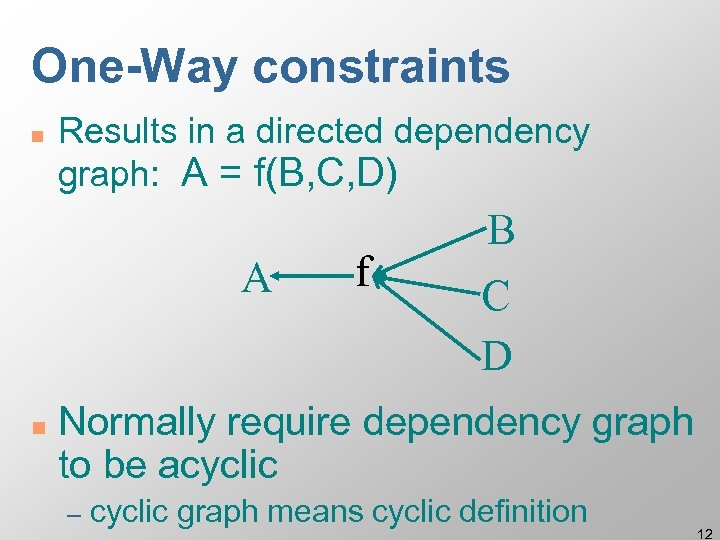One-Way constraints n Results in a directed dependency graph: A = f(B, C, D) A n f B C D Normally require dependency graph to be acyclic – cyclic graph means cyclic definition 12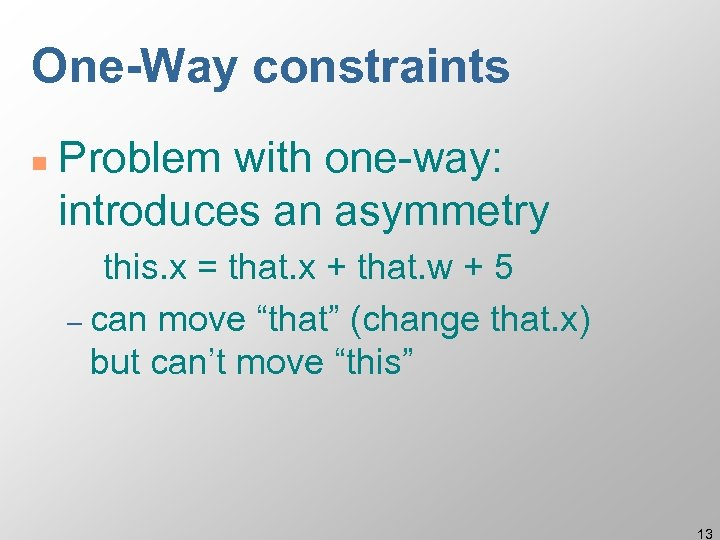One-Way constraints n Problem with one-way: introduces an asymmetry this. x = that. x + that. w + 5 – can move “that” (change that. x) but can’t move “this” 13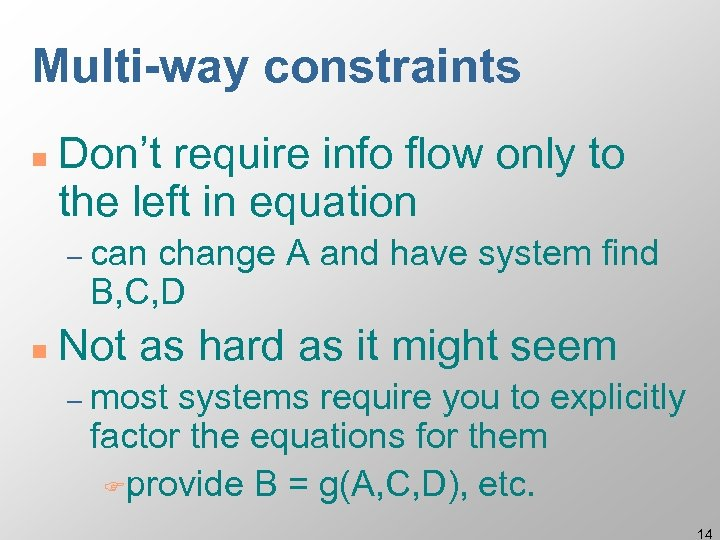Multi-way constraints n Don’t require info flow only to the left in equation – can change A and have system find B, C, D n Not as hard as it might seem – most systems require you to explicitly factor the equations for them Fprovide B = g(A, C, D), etc. 14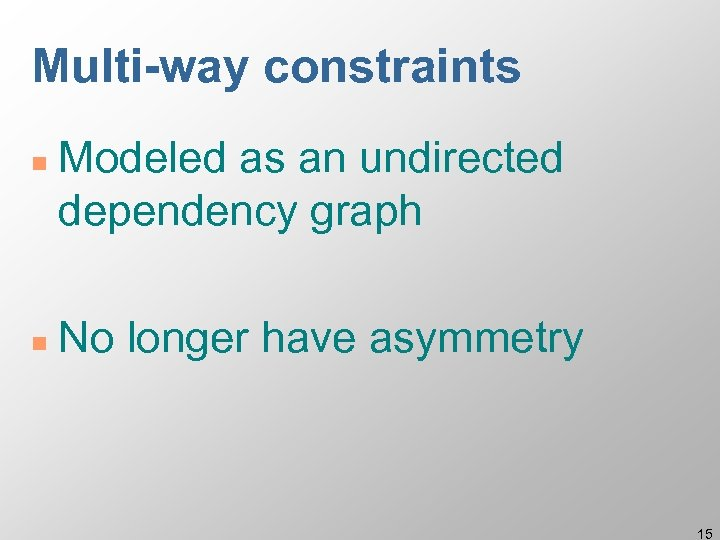Multi-way constraints n n Modeled as an undirected dependency graph No longer have asymmetry 15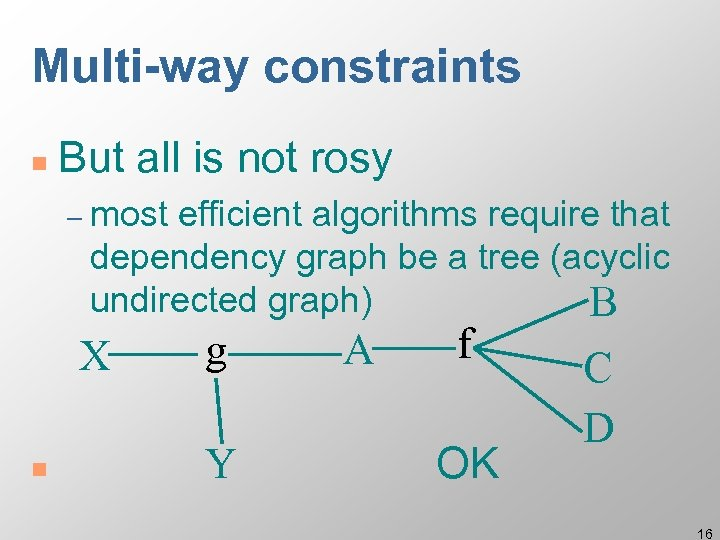Multi-way constraints n But all is not rosy – most efficient algorithms require that dependency graph be a tree (acyclic undirected graph) B X n g Y A f OK C D 16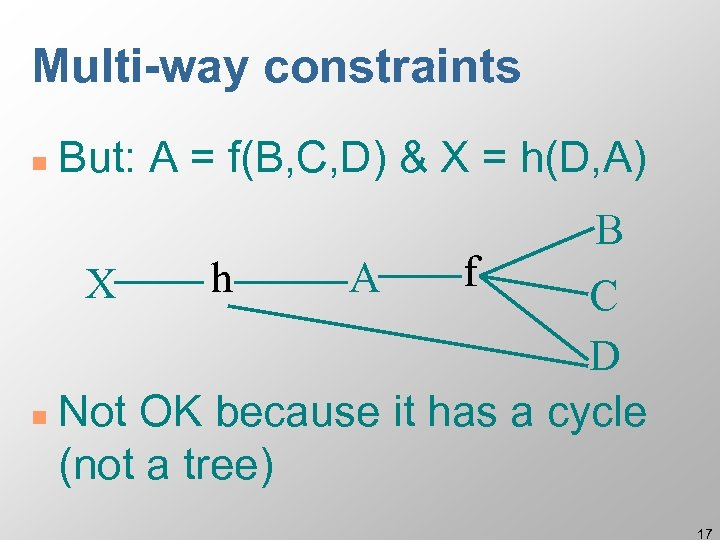Multi-way constraints n But: A = f(B, C, D) & X = h(D, A) X h A f B C D n Not OK because it has a cycle (not a tree) 17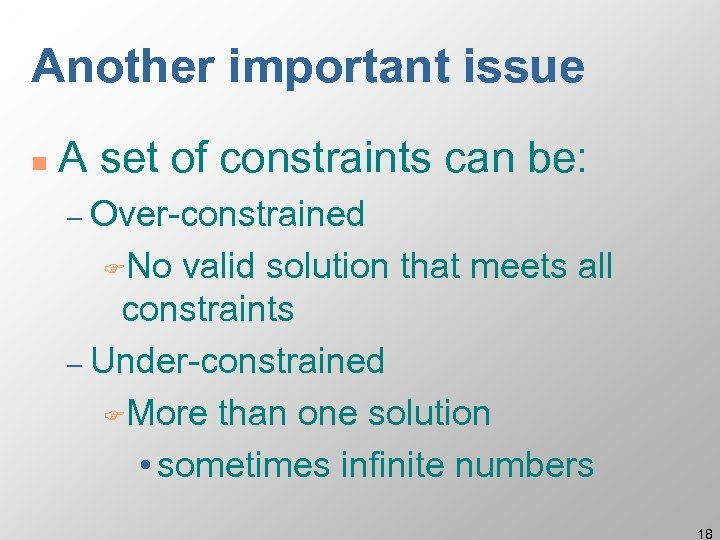Another important issue n A set of constraints can be: – Over-constrained FNo valid solution that meets all constraints – Under-constrained FMore than one solution • sometimes infinite numbers 18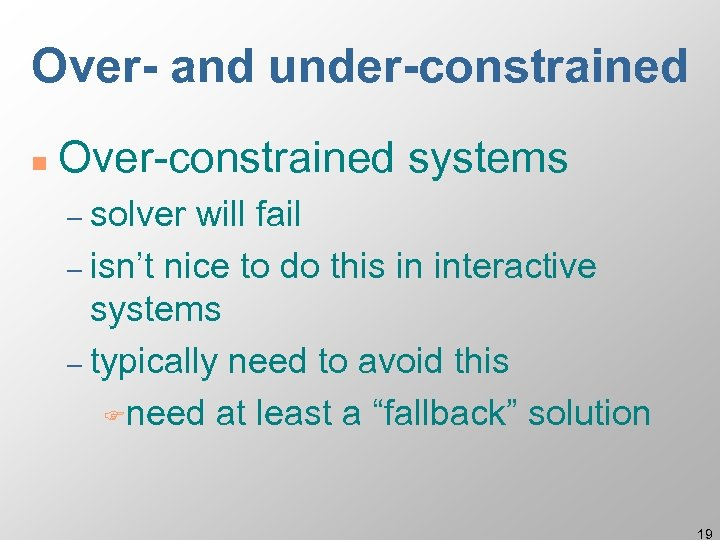Over- and under-constrained n Over-constrained systems – solver will fail – isn’t nice to do this in interactive systems – typically need to avoid this Fneed at least a “fallback” solution 19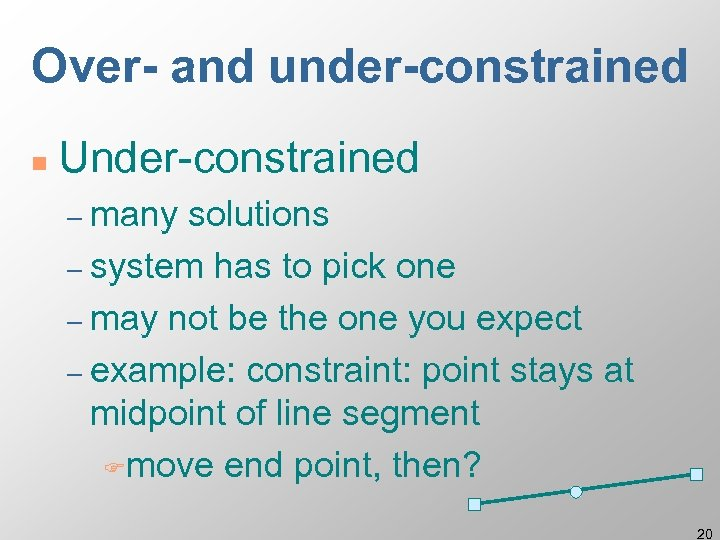Over- and under-constrained n Under-constrained – many solutions – system has to pick one – may not be the one you expect – example: constraint: point stays at midpoint of line segment Fmove end point, then? 20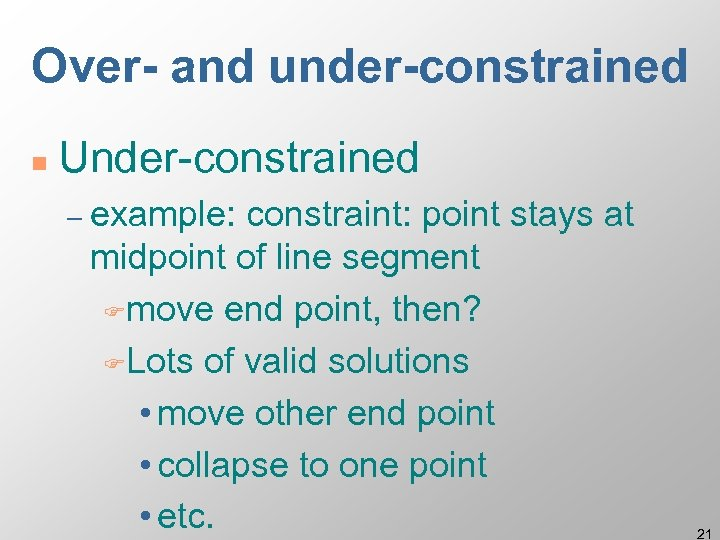Over- and under-constrained n Under-constrained – example: constraint: point stays at midpoint of line segment Fmove end point, then? FLots of valid solutions • move other end point • collapse to one point • etc. 21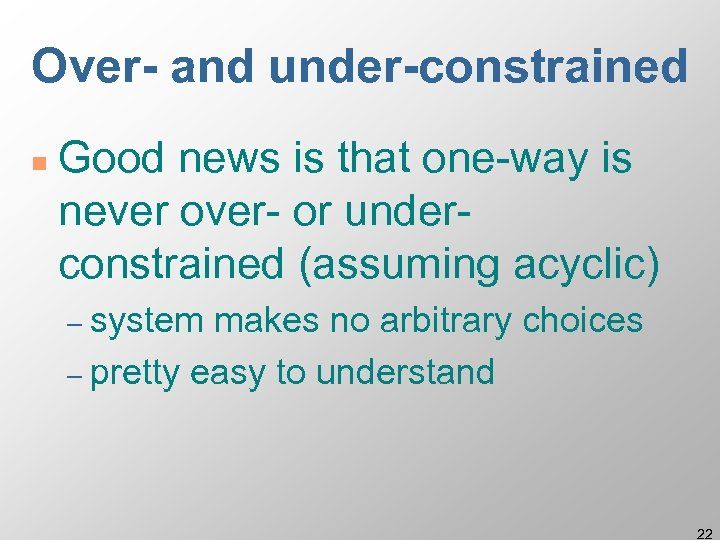Over- and under-constrained n Good news is that one-way is never over- or underconstrained (assuming acyclic) – system makes no arbitrary choices – pretty easy to understand 22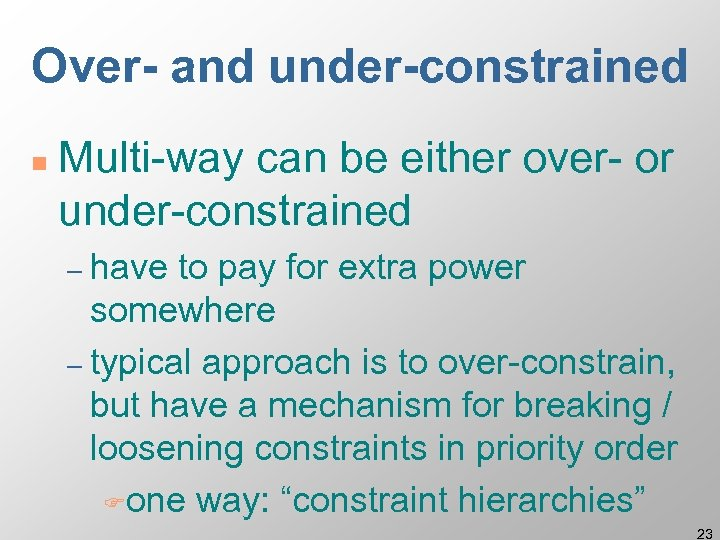Over- and under-constrained n Multi-way can be either over- or under-constrained – have to pay for extra power somewhere – typical approach is to over-constrain, but have a mechanism for breaking / loosening constraints in priority order Fone way: “constraint hierarchies” 23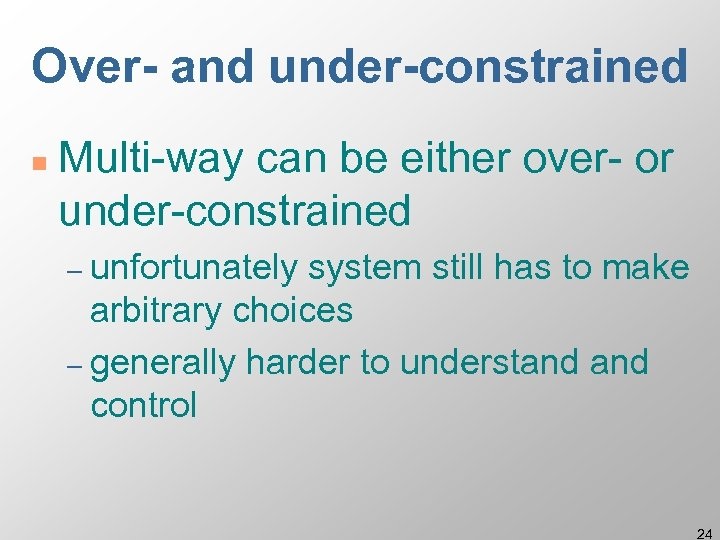Over- and under-constrained n Multi-way can be either over- or under-constrained – unfortunately system still has to make arbitrary choices – generally harder to understand control 24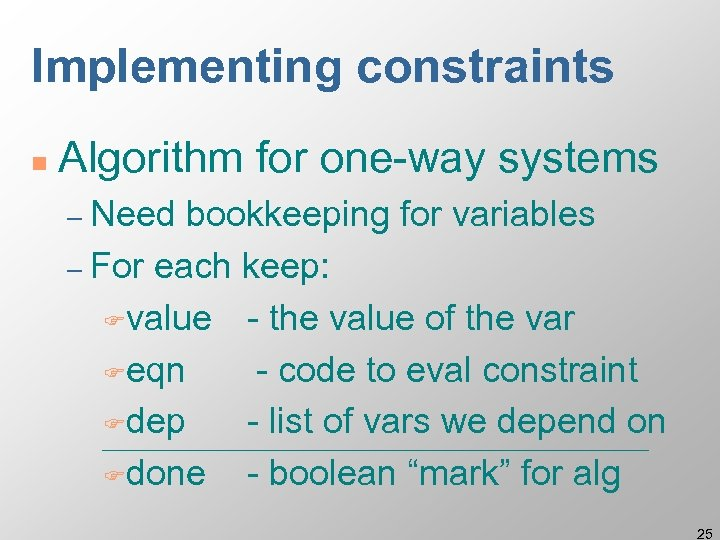Implementing constraints n Algorithm for one-way systems – Need bookkeeping for variables – For each keep: Fvalue - the value of the var Feqn - code to eval constraint Fdep - list of vars we depend on Fdone - boolean “mark” for alg 25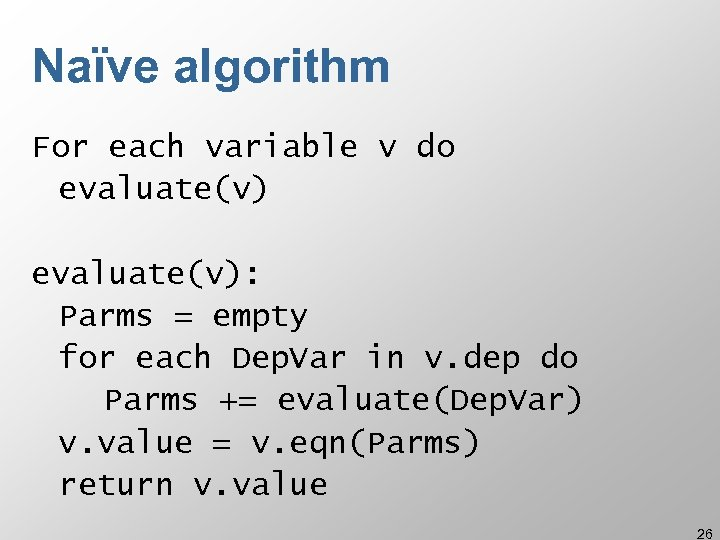Naïve algorithm For each variable v do evaluate(v): Parms = empty for each Dep. Var in v. dep do Parms += evaluate(Dep. Var) v. value = v. eqn(Parms) return v. value 26Why is this not a good plan? 27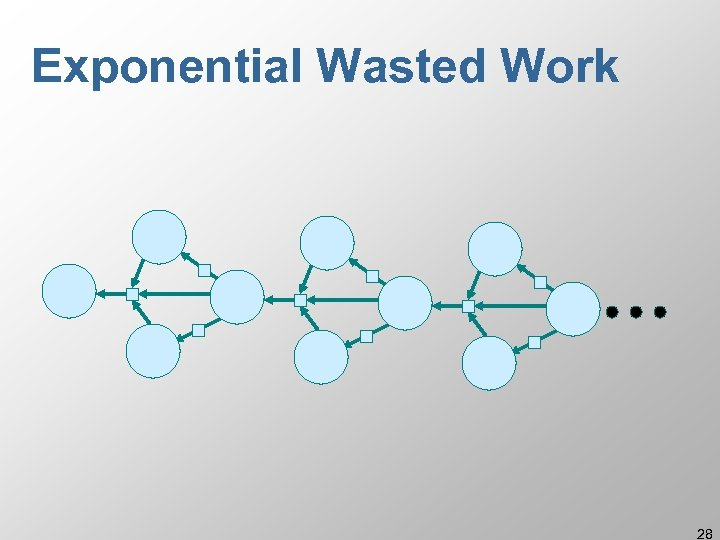Exponential Wasted Work 28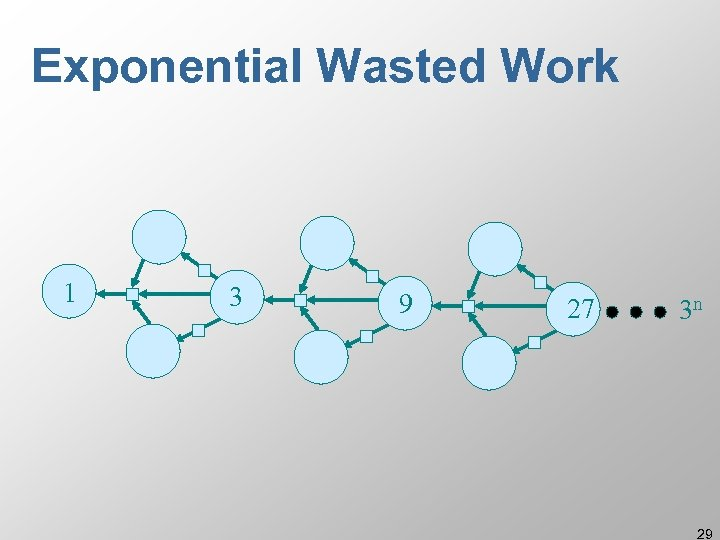Exponential Wasted Work 1 3 9 27 3 n 29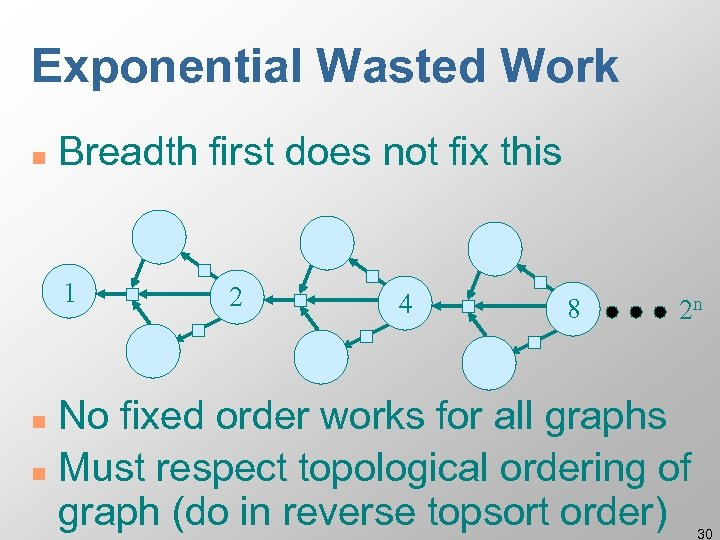Exponential Wasted Work n Breadth first does not fix this 1 2 4 8 2 n No fixed order works for all graphs n Must respect topological ordering of graph (do in reverse topsort order) n 30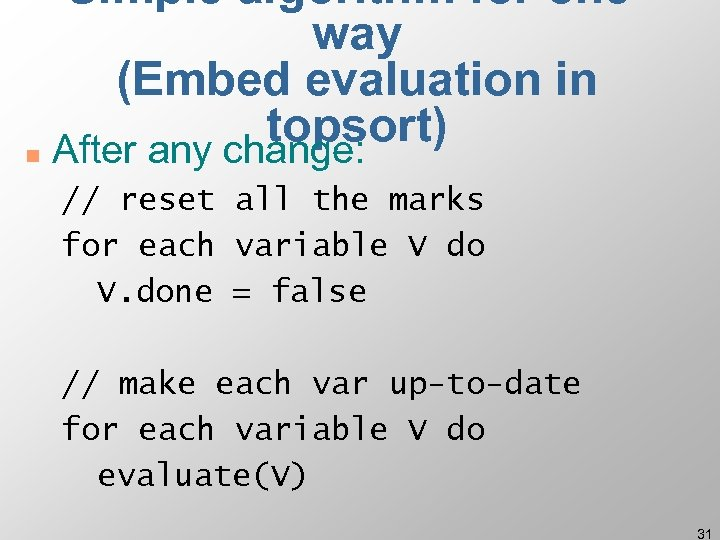Simple algorithm for oneway (Embed evaluation in topsort) n After any change: // reset all the marks for each variable V do V. done = false // make each var up-to-date for each variable V do evaluate(V) 31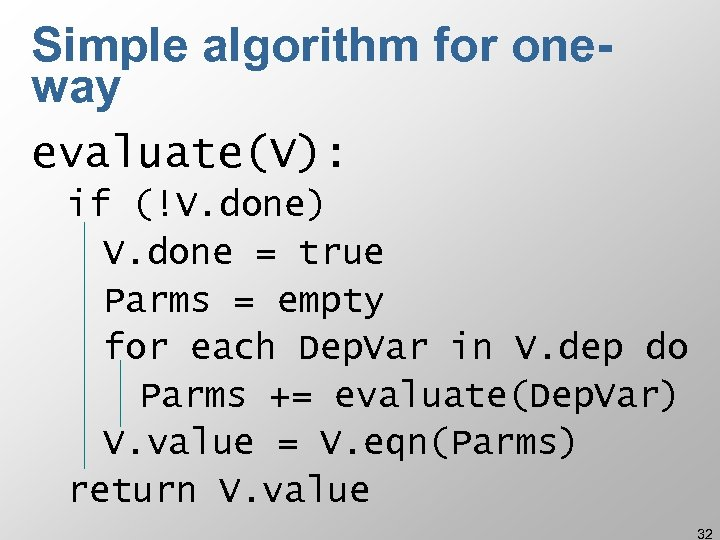Simple algorithm for oneway evaluate(V): if (!V. done) V. done = true Parms = empty for each Dep. Var in V. dep do Parms += evaluate(Dep. Var) V. value = V. eqn(Parms) return V. value 32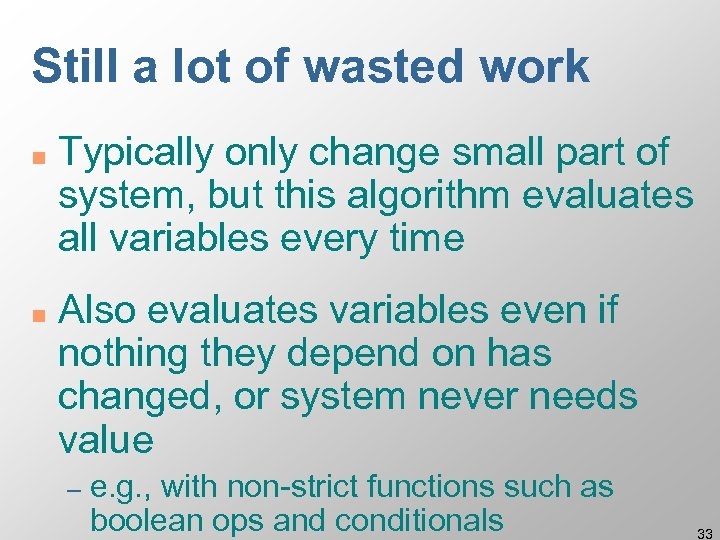Still a lot of wasted work n n Typically only change small part of system, but this algorithm evaluates all variables every time Also evaluates variables even if nothing they depend on has changed, or system never needs value – e. g. , with non-strict functions such as boolean ops and conditionals 33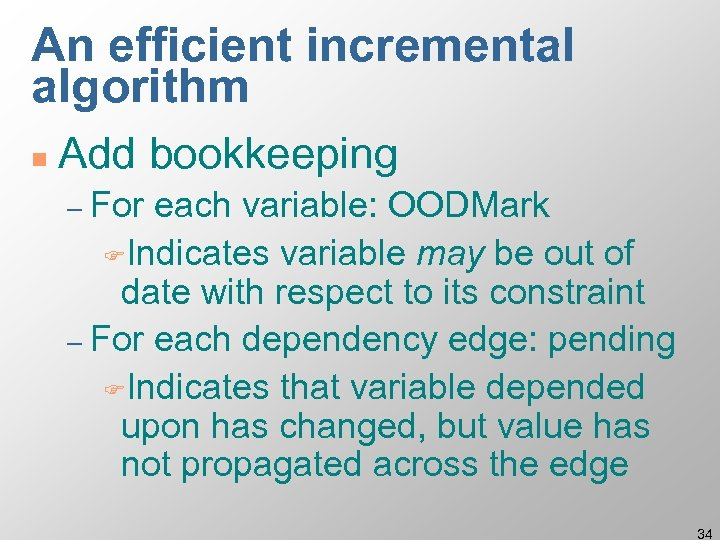An efficient incremental algorithm n Add bookkeeping – For each variable: OODMark FIndicates variable may be out of date with respect to its constraint – For each dependency edge: pending FIndicates that variable depended upon has changed, but value has not propagated across the edge 34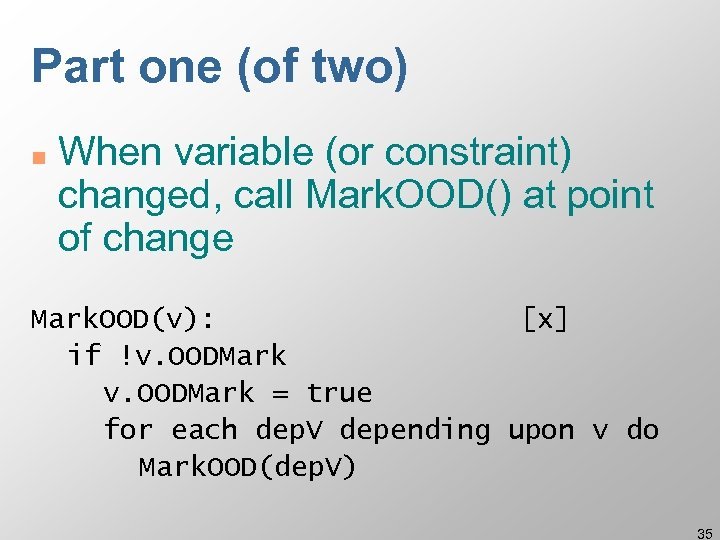Part one (of two) n When variable (or constraint) changed, call Mark. OOD() at point of change Mark. OOD(v): [x] if !v. OODMark = true for each dep. V depending upon v do Mark. OOD(dep. V) 35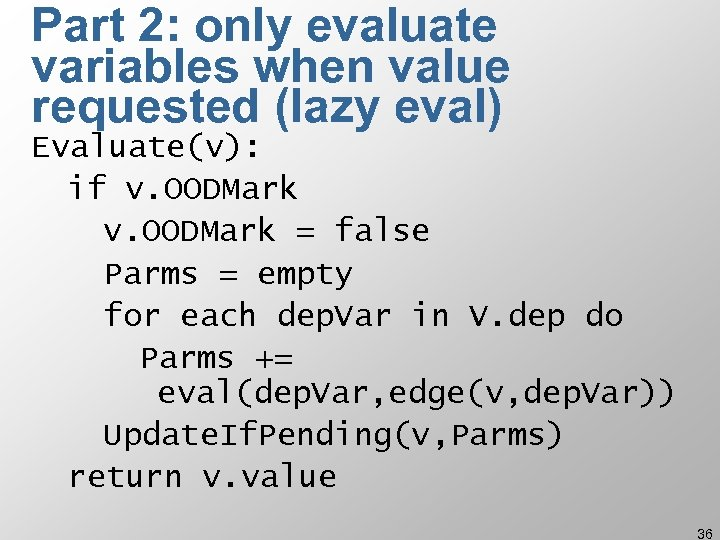Part 2: only evaluate variables when value requested (lazy eval) Evaluate(v): if v. OODMark = false Parms = empty for each dep. Var in V. dep do Parms += eval(dep. Var, edge(v, dep. Var)) Update. If. Pending(v, Parms) return v. value 36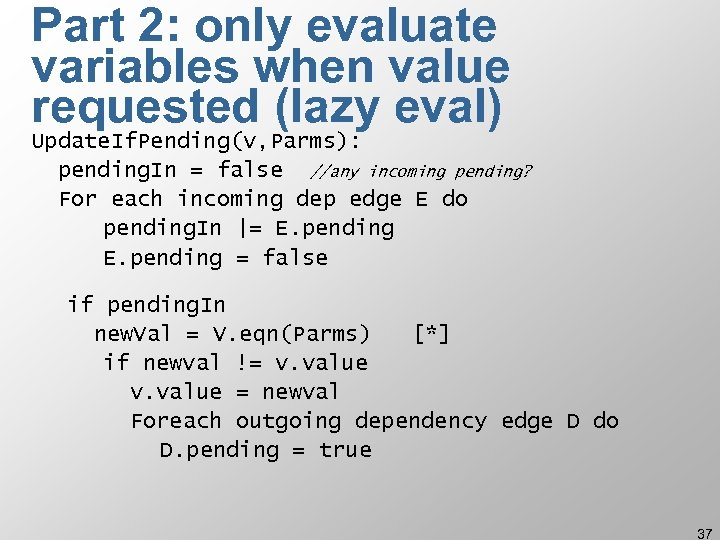Part 2: only evaluate variables when value requested (lazy eval) Update. If. Pending(v, Parms): pending. In = false //any incoming pending? For each incoming dep edge E do pending. In |= E. pending = false if pending. In new. Val = V. eqn(Parms) [*] if newval != v. value = newval Foreach outgoing dependency edge D do D. pending = true 37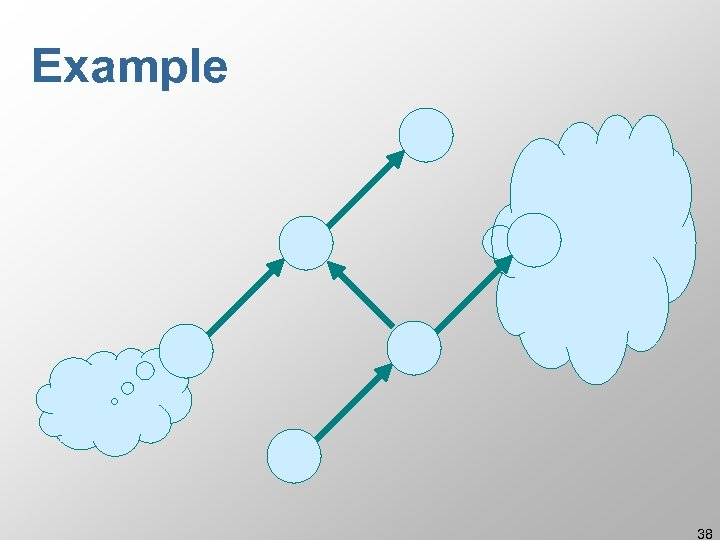Example 38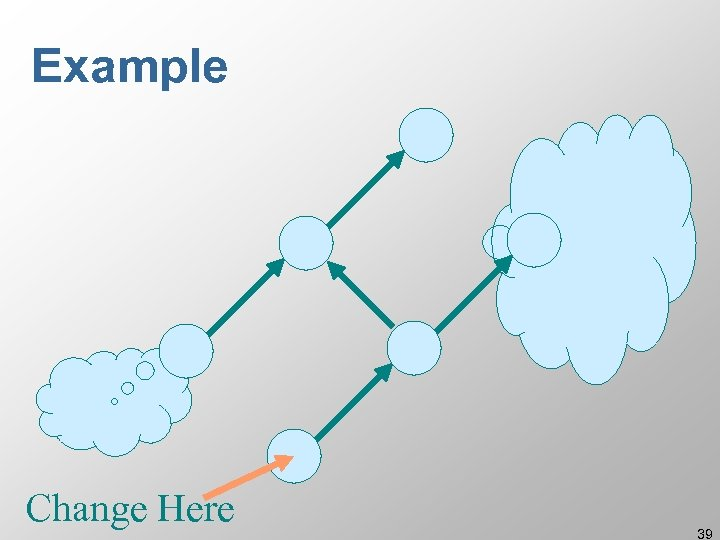Example Change Here 39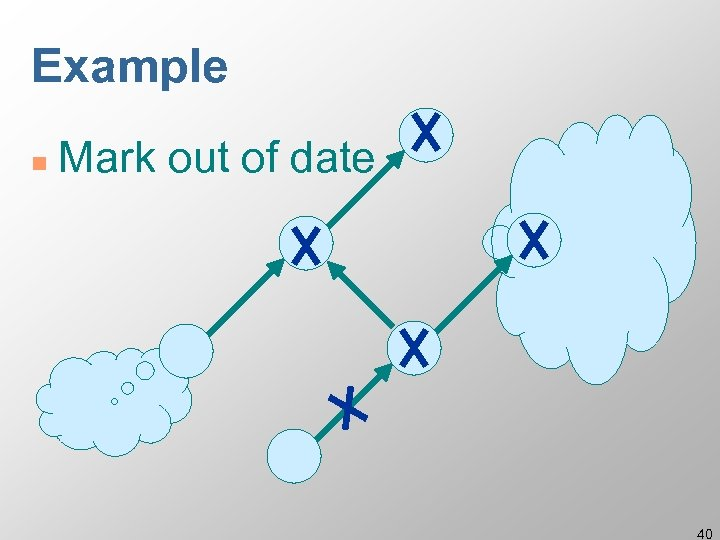Example n Mark out of date 40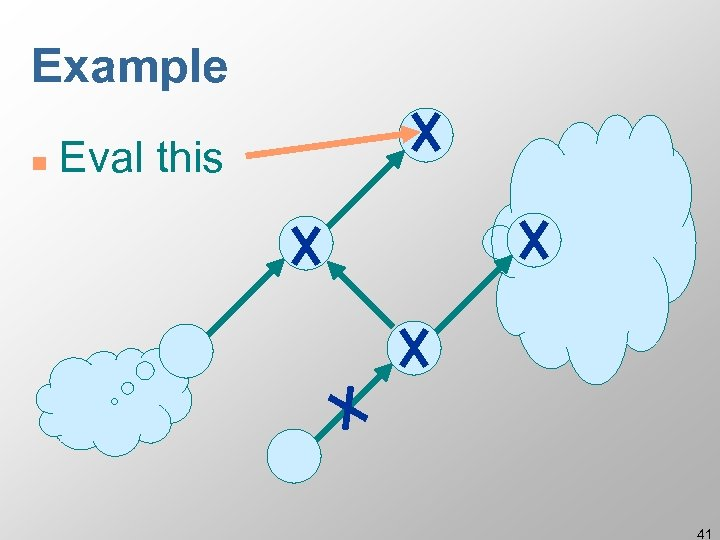Example n Eval this 41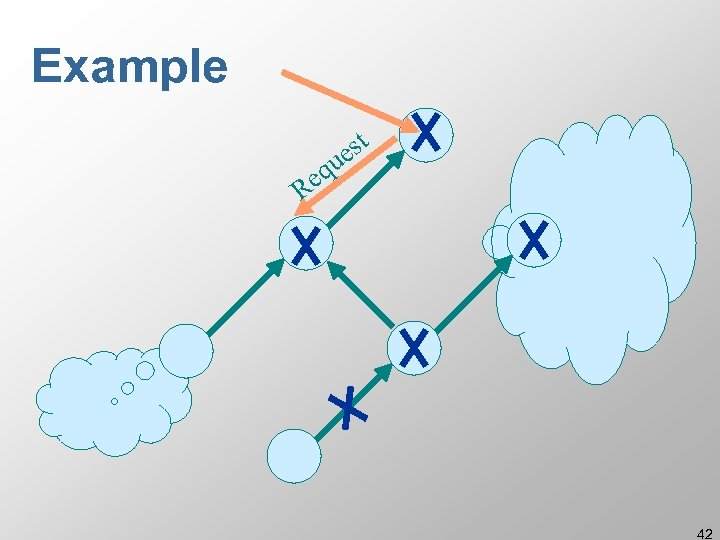Example t es u eq R 42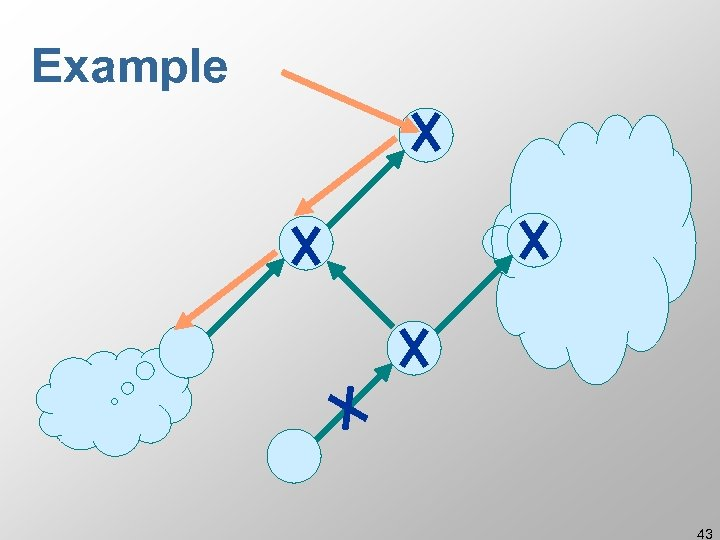Example 43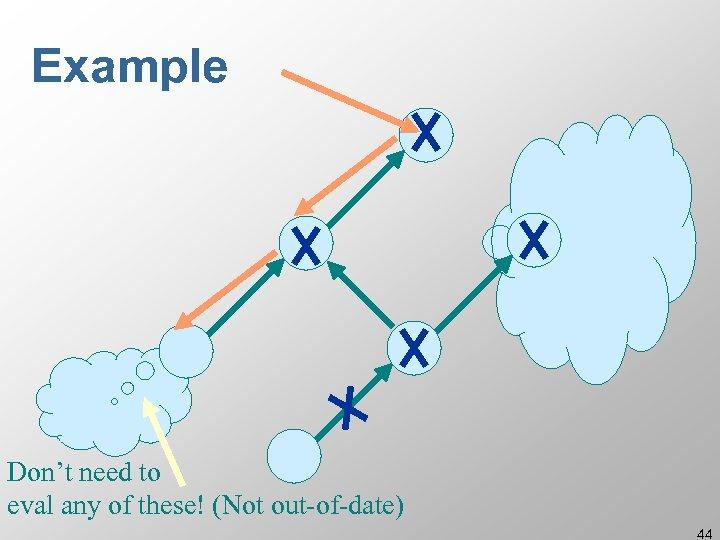Example Don’t need to eval any of these! (Not out-of-date) 44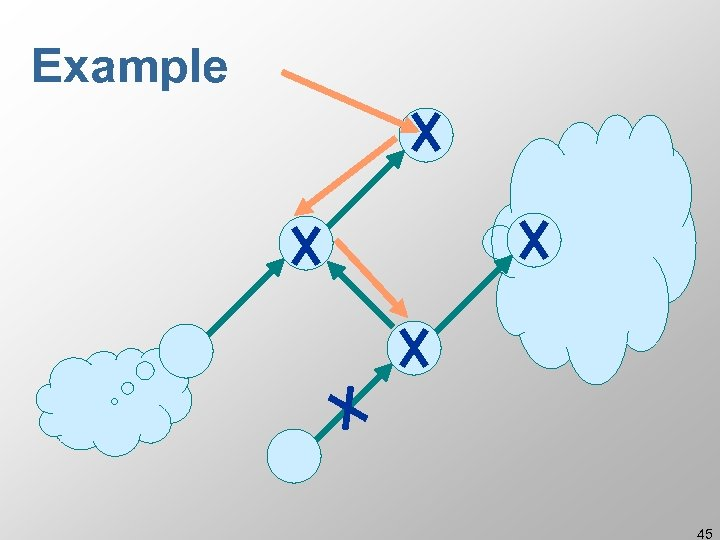Example 45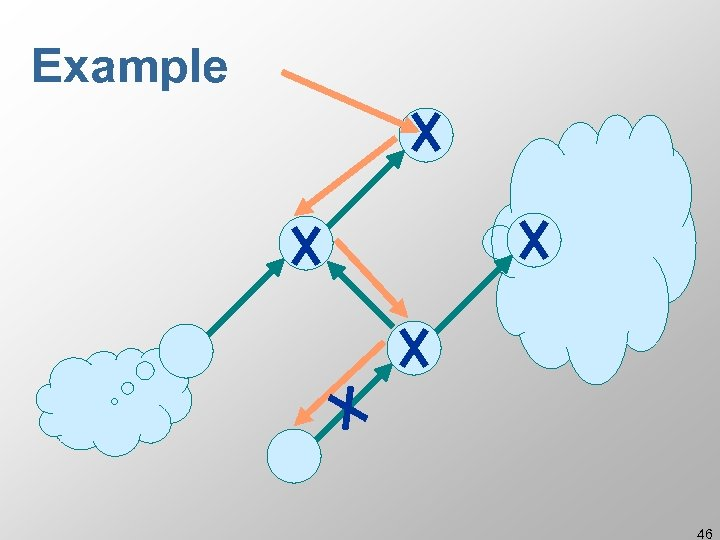Example 46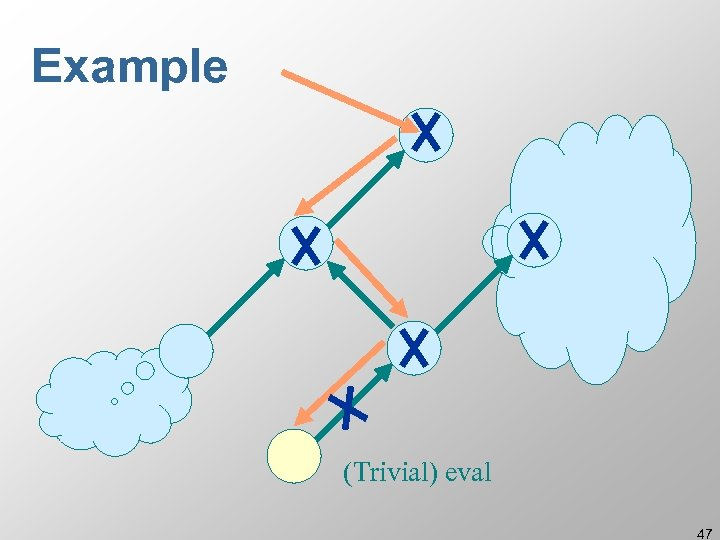Example (Trivial) eval 47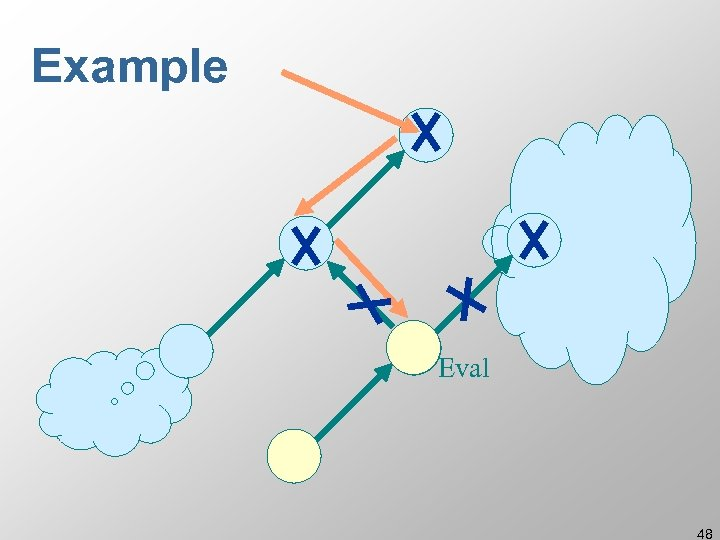Example Eval 48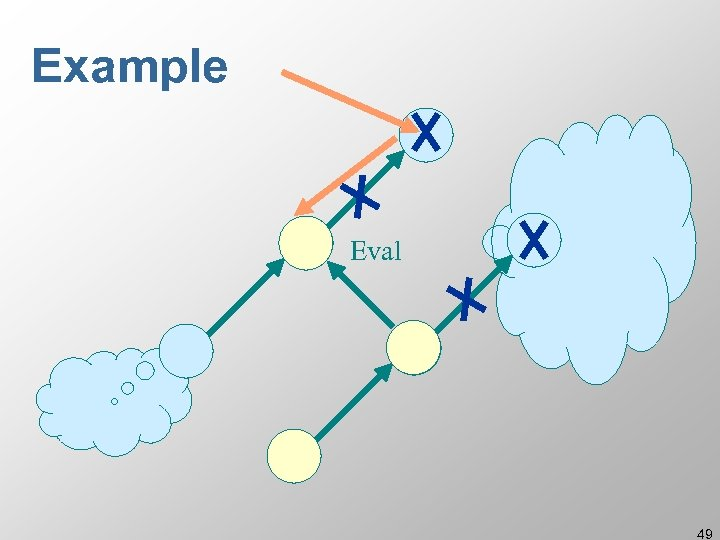Example Eval 49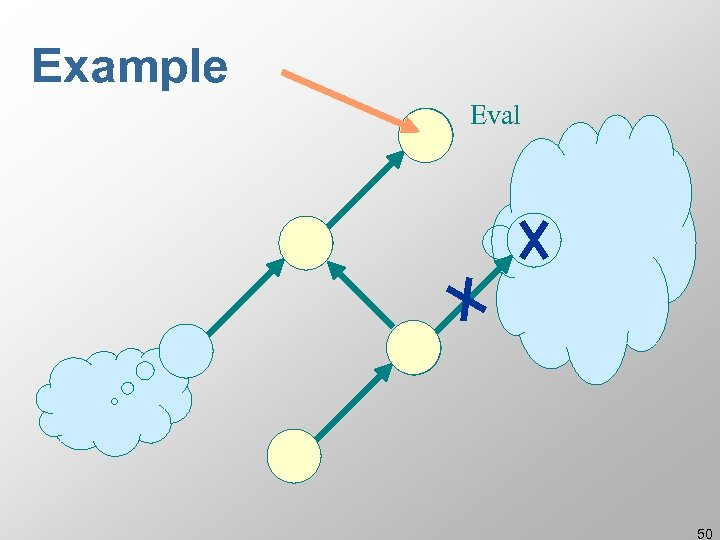Example Eval 50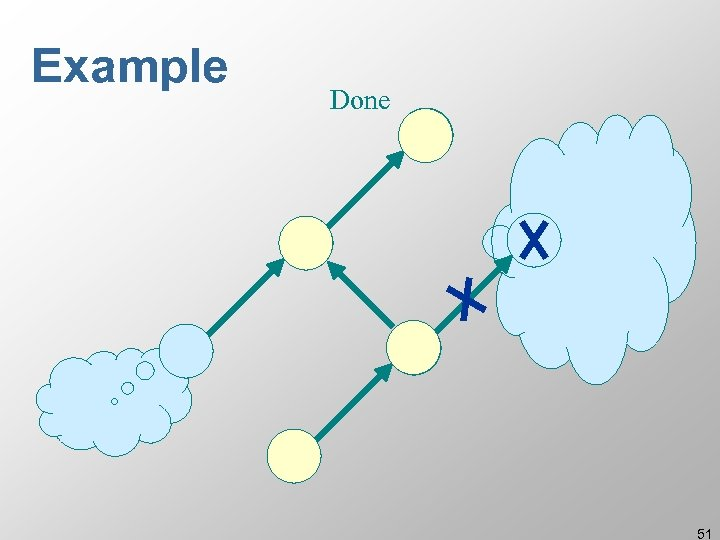Example Done 51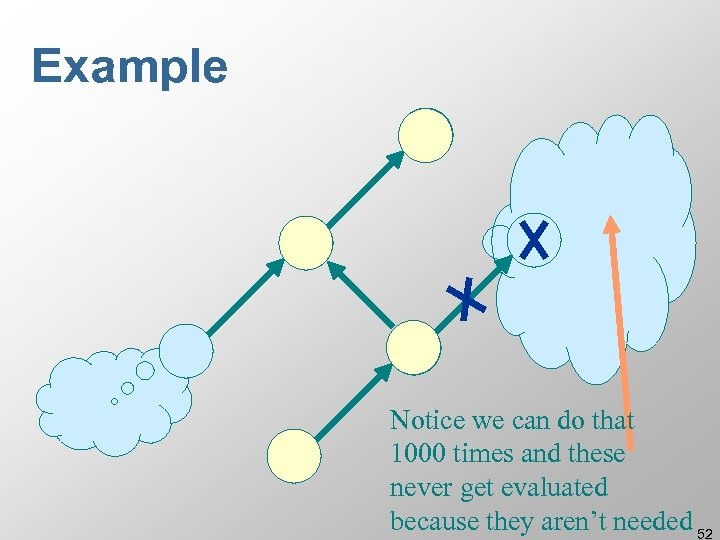Example Notice we can do that 1000 times and these never get evaluated because they aren’t needed 52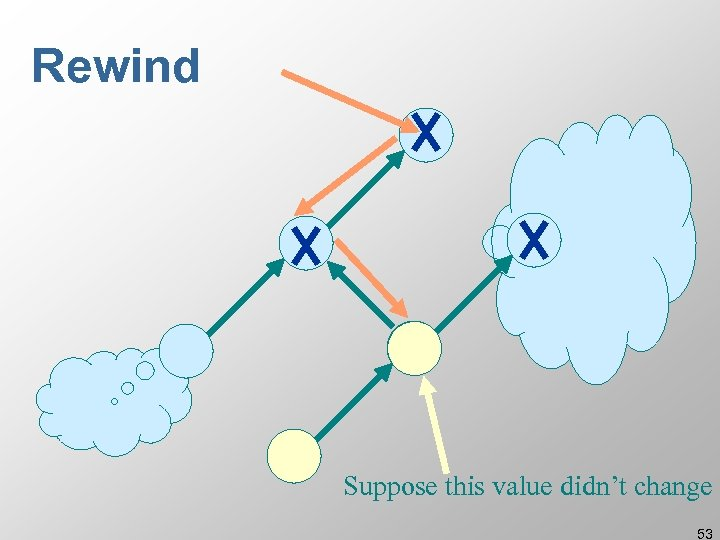Rewind Suppose this value didn’t change 53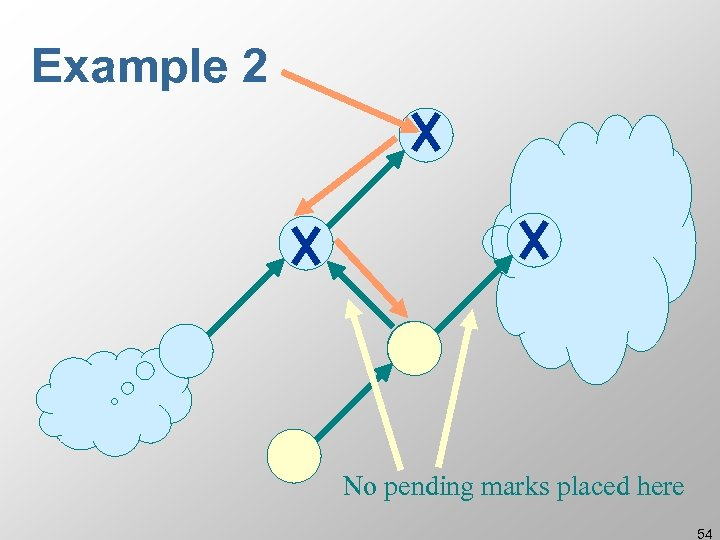Example 2 No pending marks placed here 54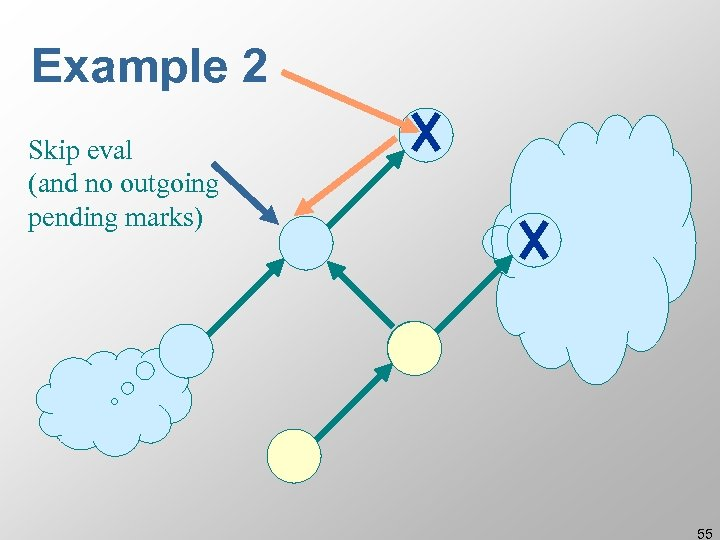Example 2 Skip eval (and no outgoing pending marks) 55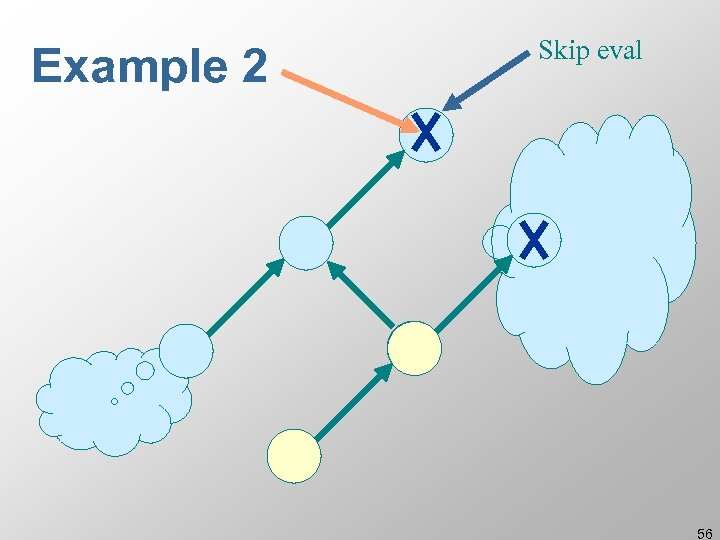Example 2 Skip eval 56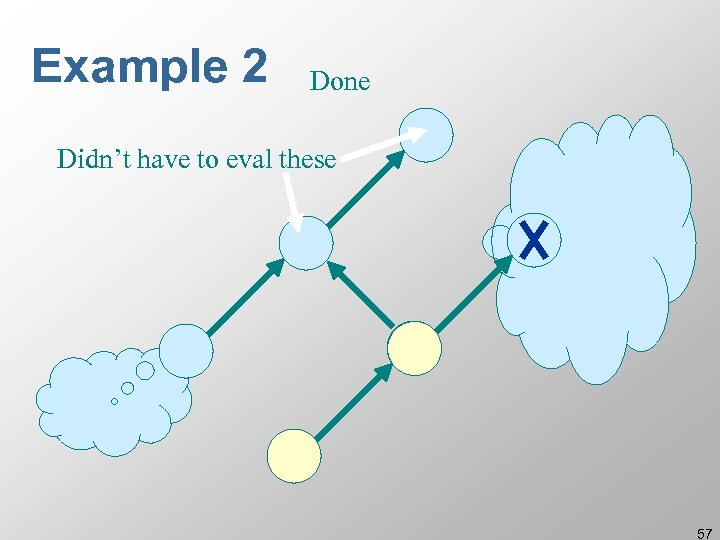Example 2 Done Didn’t have to eval these 57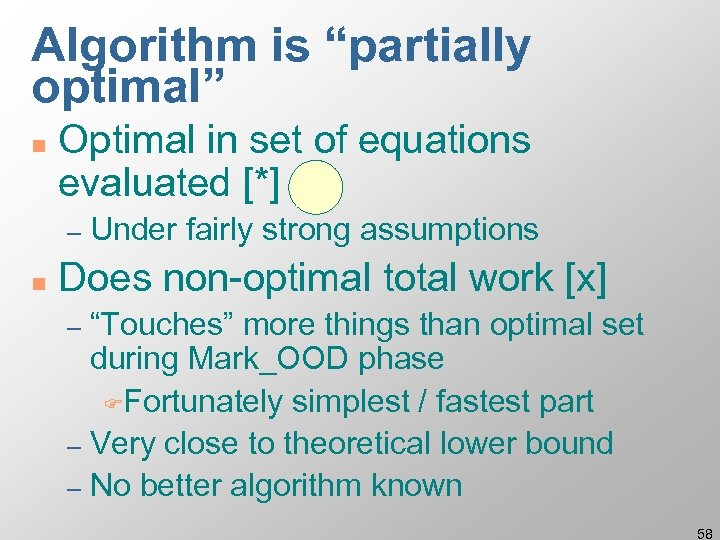Algorithm is “partially optimal” n Optimal in set of equations evaluated [*] – n Under fairly strong assumptions Does non-optimal total work [x] “Touches” more things than optimal set during Mark_OOD phase FFortunately simplest / fastest part – Very close to theoretical lower bound – No better algorithm known – 58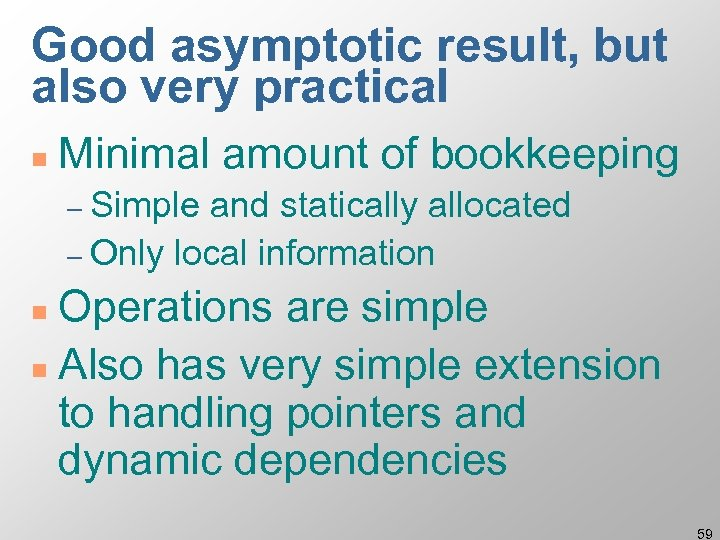Good asymptotic result, but also very practical n Minimal amount of bookkeeping – Simple and statically allocated – Only local information Operations are simple n Also has very simple extension to handling pointers and dynamic dependencies n 59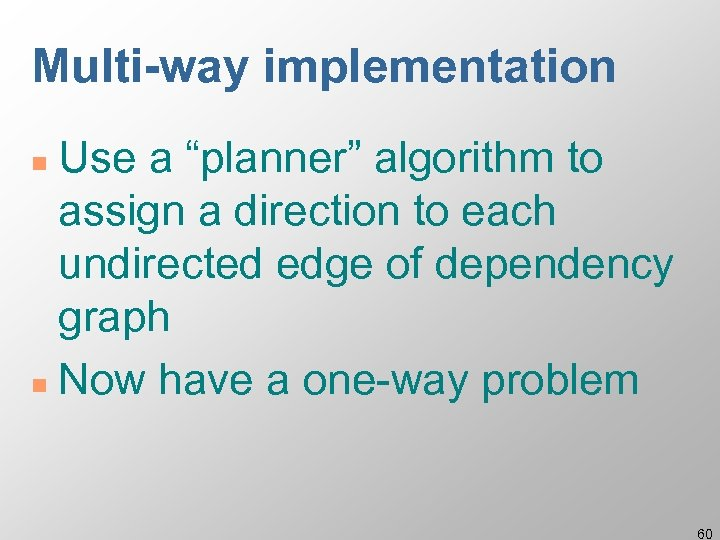Multi-way implementation Use a “planner” algorithm to assign a direction to each undirected edge of dependency graph n Now have a one-way problem n 60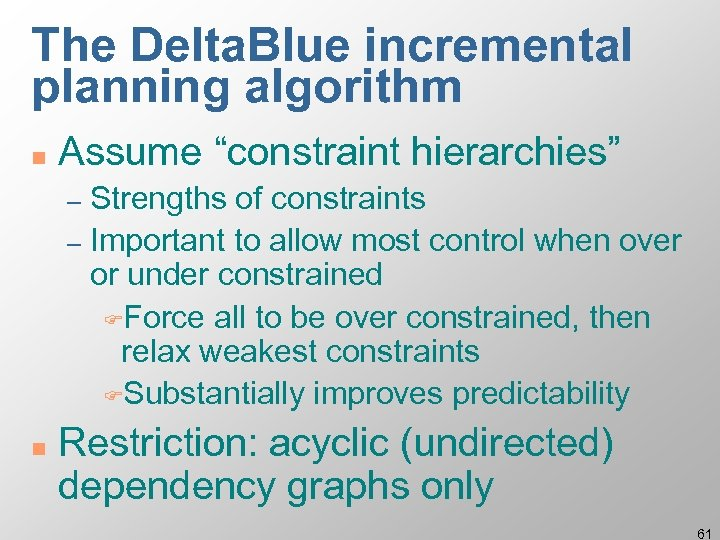The Delta. Blue incremental planning algorithm n Assume “constraint hierarchies” Strengths of constraints – Important to allow most control when over or under constrained FForce all to be over constrained, then relax weakest constraints FSubstantially improves predictability – n Restriction: acyclic (undirected) dependency graphs only 61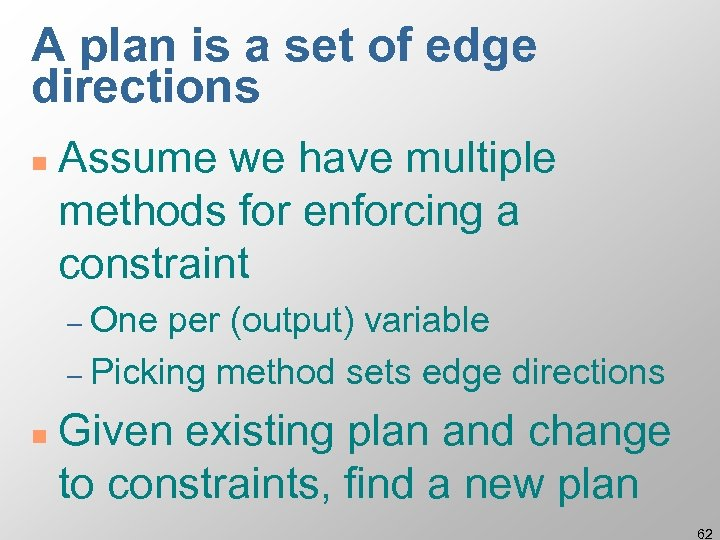A plan is a set of edge directions n Assume we have multiple methods for enforcing a constraint – One per (output) variable – Picking method sets edge directions n Given existing plan and change to constraints, find a new plan 62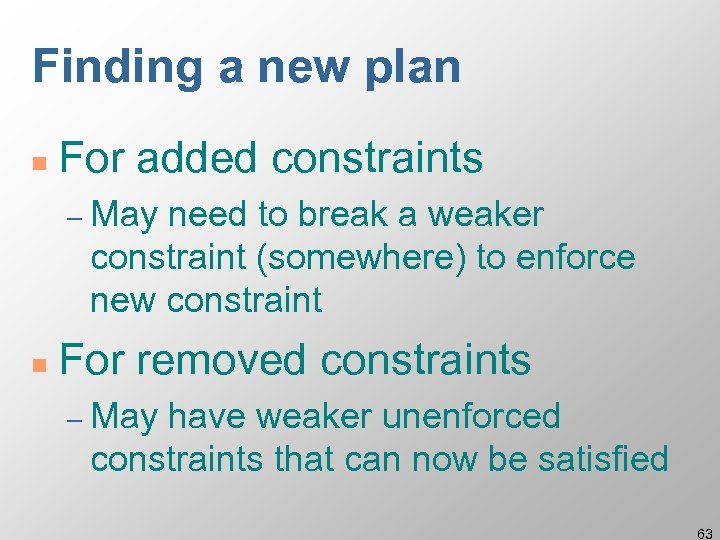Finding a new plan n For added constraints – May need to break a weaker constraint (somewhere) to enforce new constraint n For removed constraints – May have weaker unenforced constraints that can now be satisfied 63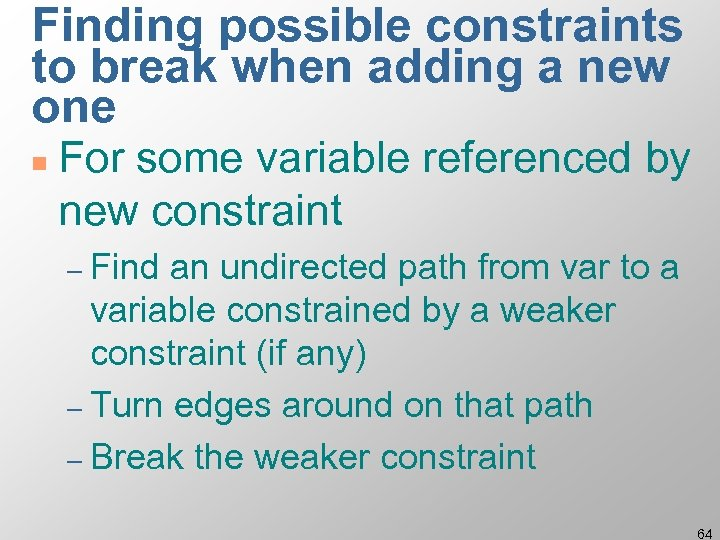Finding possible constraints to break when adding a new one n For some variable referenced by new constraint – Find an undirected path from var to a variable constrained by a weaker constraint (if any) – Turn edges around on that path – Break the weaker constraint 64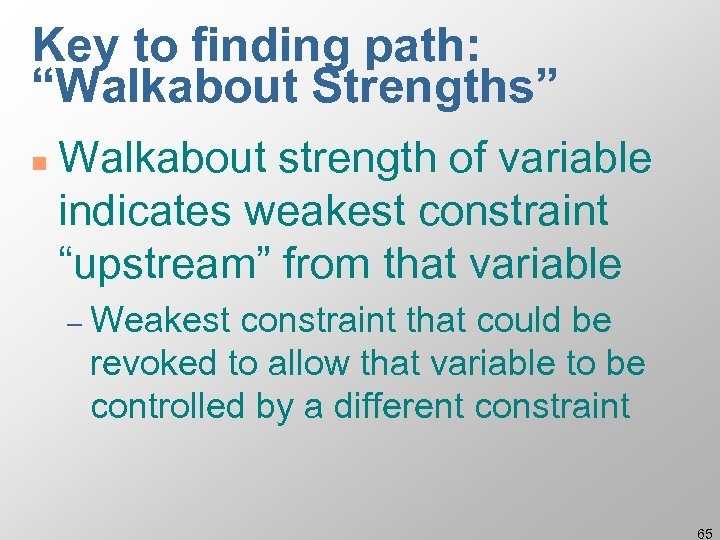Key to finding path: “Walkabout Strengths” n Walkabout strength of variable indicates weakest constraint “upstream” from that variable – Weakest constraint that could be revoked to allow that variable to be controlled by a different constraint 65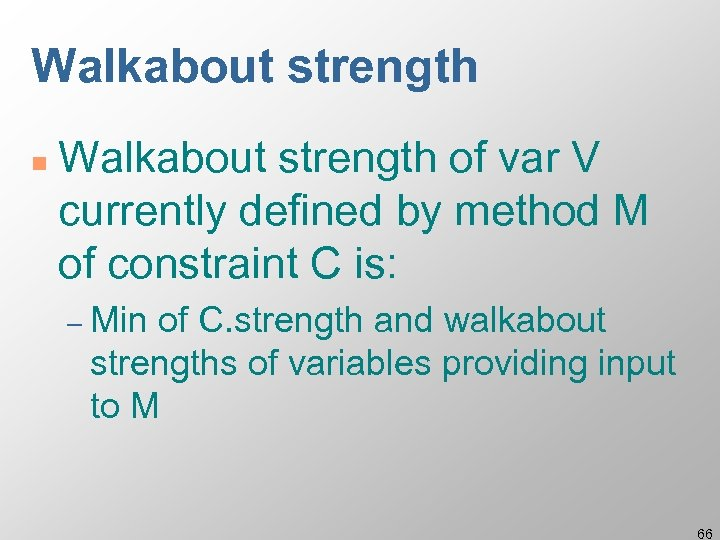Walkabout strength n Walkabout strength of var V currently defined by method M of constraint C is: – Min of C. strength and walkabout strengths of variables providing input to M 66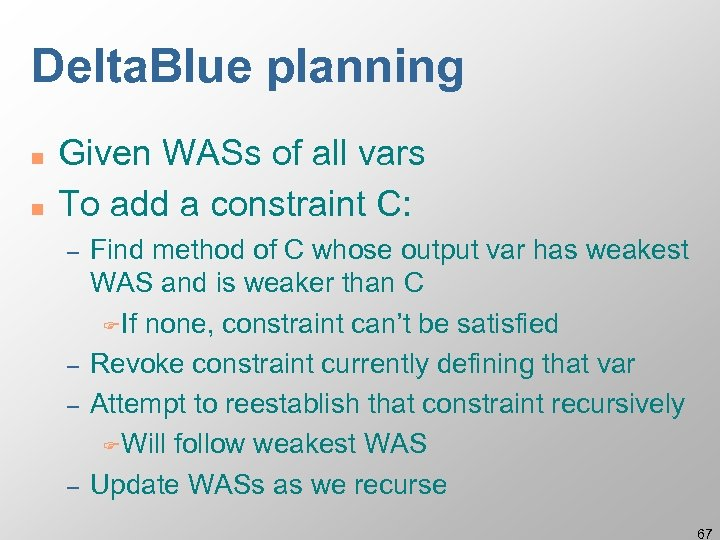Delta. Blue planning n n Given WASs of all vars To add a constraint C: – – Find method of C whose output var has weakest WAS and is weaker than C FIf none, constraint can’t be satisfied Revoke constraint currently defining that var Attempt to reestablish that constraint recursively FWill follow weakest WAS Update WASs as we recurse 67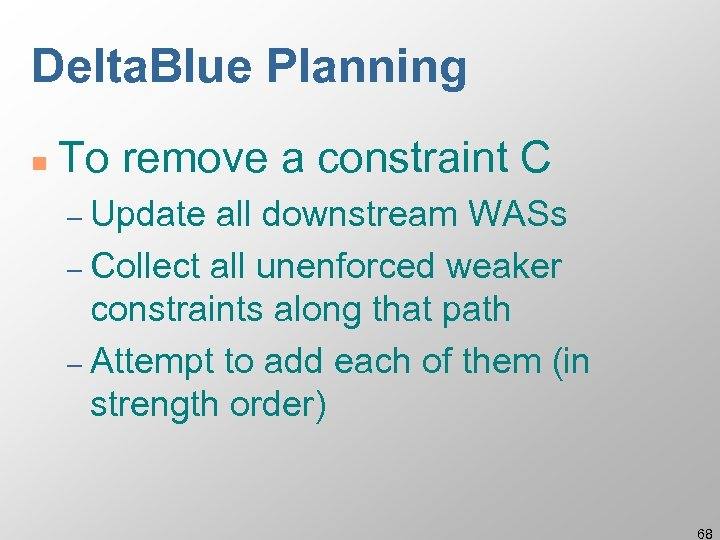Delta. Blue Planning n To remove a constraint C – Update all downstream WASs – Collect all unenforced weaker constraints along that path – Attempt to add each of them (in strength order) 68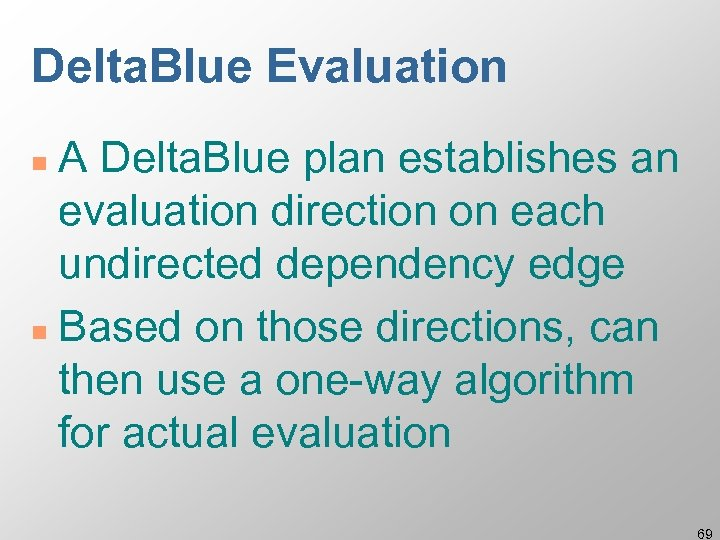Delta. Blue Evaluation A Delta. Blue plan establishes an evaluation direction on each undirected dependency edge n Based on those directions, can then use a one-way algorithm for actual evaluation n 69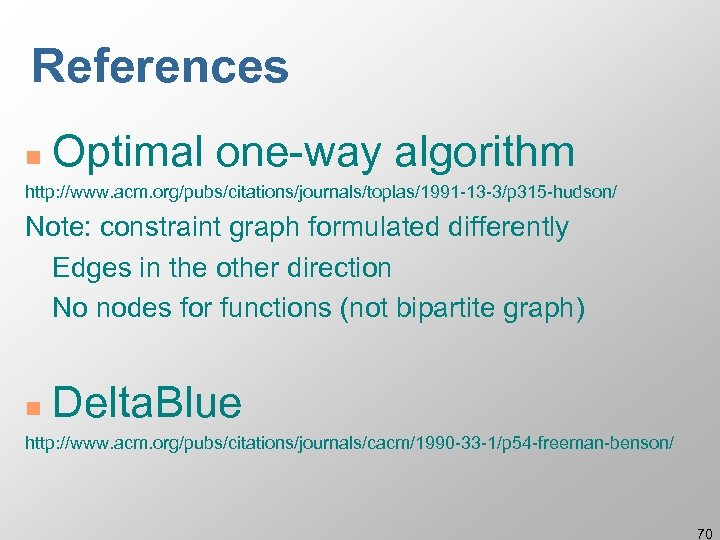References n Optimal one-way algorithm http: //www. acm. org/pubs/citations/journals/toplas/1991 -13 -3/p 315 -hudson/ Note: constraint graph formulated differently Edges in the other direction No nodes for functions (not bipartite graph) n Delta. Blue http: //www. acm. org/pubs/citations/journals/cacm/1990 -33 -1/p 54 -freeman-benson/ 7071